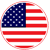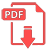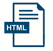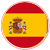# Home | Mathematics | Number Systems

## Perform hex, decimal, octal, binary math

Denary - the Denary number system (decimal) is a base 10 number system used by people with 10 unique digits 0 to 9
Octal- the Octal numeral system (Oct) is a base 8 number system that use the digits 0 to 7
Hexadecimal- the Hexadecimal number system (Hex) is a base 16 number system using number 0 - 9 and letters A - F
Binary - the Binary number system (Bin) is a base 2 number system using number 1 and 0EnglishSpanish